### 8.4.4.3 Navigation function in the sense of Rimon-Koditschek

Now consider constructing a navigation function from which a vector field can be derived that satisfies constraints motivated by the acceleration-based control model, (8.47). As usual, the definition of a navigation function begins with the consideration of a potential function,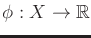. What properties does a potential function need to have so that it may be considered as a navigation function as defined in Section 8.4.1 and also yield a vector field that satisfies an acceleration bound? Sufficient conditions will be given that imply that a potential function will be a navigation function that satisfies the bound.

To give the conditions, it will first be important to characterize extrema of multivariate functions. Recall from basic calculus that a function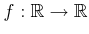has a critical point when the first derivative is zero. At such points, the sign of the second derivative indicates whether the critical point is a minimum or maximum. These ideas can be generalized to higher dimensions. A critical point of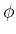is one for which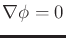. The Hessian ofis defined as the matrix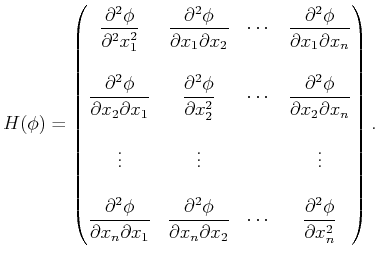(8.48)

At each critical point, the Hessian gives some information about the extremum. If the rank of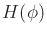atis, then the Hessian indicates the kind of extremum. If (8.48) is positive definite,8.13 then theachieves a local minimum at. If (8.48) is negative definite,8.14 then theachieves a local maximum at. In all other cases,is a saddle point. If the rank ofatis less than, then the Hessian is degenerate. In this case the Hessian cannot classify the type of extremum. An example of this occurs whenlies in a plateau (there is no direction in whichincreases or decreases. Such behavior is obviously bad for a potential function because the local operator would not be able to select a direction.

Suppose that the navigation function is required to be smooth, to ensure the existence of a gradient at every point. This enables gradient descent to be performed. Ifis not contractible, then it turns out there must exist some critical points other than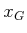at which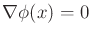. The critical points can even be used to infer the topology of, which is the basic idea in the subject of Morse theory [701,234]. Unfortunately, this implies that there does not exist a solution navigation function for such spaces because the definition in Section 8.4.1 required that the integral curve from any state that can reachmust reach it using the vector field derived from the navigation function. If the initial state is a critical point, the integral curve is constant (the state remains at the critical point). Therefore, under the smoothness constraint, the definition of a navigation function should be modified to allow critical points at a small number of places (only on a set that has measure zero). It is furthermore required that the set of states from which the integral curves arrive at each critical point (i.e., the domain of attraction of each critical point) has measure zero. From all possible initial states, except from a set of measure zero, the integral curves must reach, if it is reachable. This is ensured in the following definition.

A functionis called a navigation function in the sense of Rimon-Koditschek if :

1. It is smooth (or at least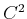).
2. Among all values on the connected component ofthat contains, there is only one local minimum, which is at.8.15
3. It is maximal and constant on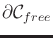, the boundary of.
4. It is a Morse function , which means that at each critical point(i.e.,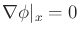), the Hessian ofis not degenerate.8.16 Such functions are known to exist on any smooth manifold.
Ifis smooth in the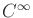sense, then by Sard's Theorem  the set of critical points has measure zero.

Methods for constructing navigation functions are outlined in  for a general family of problems in whichhas a semi-algebraic description. The basic idea is to start with simple shapes over which a navigation function can be easily defined. One example of this is a spherical subset of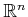, which contains spherical obstacles. A set of distorting transformations is then developed to adapt the navigation functions to other shapes while ensuring that the four properties above are maintained. One such transformation extends a ball into any visibility region (in the sense defined in Section 8.4.3). This is achieved by smoothly stretching out the ball into the shape of the visibility region. (Such regions are sometimes called star-shaped.) The transformations given in  can be combined to define navigation functions for a large family of configuration spaces. The main problem is that the configuration space obstacles and the connectivity ofare represented only implicitly, which makes it difficult to correctly apply the method to complicated high-dimensional problems. One of the advantages of the approach is that proving convergence to the goal is simplified. In many cases, Lyapunov stability analysis can be performed (see Section 15.1.1).

Steven M LaValle 2020-08-14